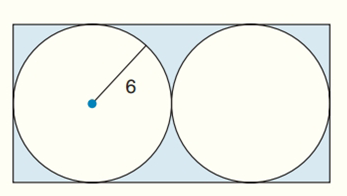Chapter 8.CR, Problem 30CRElementary Geometry For College St...

7th Edition
Alexander + 2 others
ISBN: 9781337614085

Solutions

Chapter
SectionElementary Geometry For College St...

7th Edition
Alexander + 2 others
ISBN: 9781337614085
Textbook Problem

Find the exact areas of the shaded regions in Exercises 27 to 31.Two ≅ tangent circles, inscribed in a rectangle.

To determine

To find:

The exact areas of the shaded regions.

Explanation

Calculation:

Consider the two tangent circles are shown below:

The above two tangent circles inscribed in a rectangle are congruent.

That is radius and areas of the two circles are same.

Area of the first circle A=πr2

Radius (r) = 6

A=π(6)2=36π

The total area of the two circles = Area of the first circle + Area of the second circle

=36π+36π=72π

To find the area of the rectangle:

The area A of a rectangle whose base has length b and whose altitude has length h is given by

Still sussing out bartleby?

Check out a sample textbook solution.

See a sample solution

The Solution to Your Study Problems

Bartleby provides explanations to thousands of textbook problems written by our experts, many with advanced degrees!

Get Started

Find the domain of the function f(x)=142xx2.

Precalculus: Mathematics for Calculus (Standalone Book)

(exex2)1/2

Applied Calculus for the Managerial, Life, and Social Sciences: A Brief Approach

True or False: is a convergent series.

Study Guide for Stewart's Multivariable Calculus, 8th

Rectangular coordinates of the point with polar coordinates are: (−1, 0) (0, 1) (0, −1) (1, 0)

Study Guide for Stewart's Single Variable Calculus: Early Transcendentals, 8th